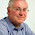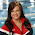## Pages

### Meriden Tugo and Kozy Companies

Price: \$4.99BE18-8 Meriden Company has a unit selling price of \$520, variable costs per unit of
\$286, and fixed costs of \$187,200. Compute the break-even point in units using (a) the
mathematical equation and (b) contribution margin per unit.

BE18-10 For Turgo Company, variable costs are 60% of sales, and fixed costs are
\$195,000. Management’s net income goal is \$75,000. Compute the required sales in dollars
needed to achieve management’s target net income of \$75,000. (Use the contribution
margin approach.)

BE18-11 For Kozy Company, actual sales are \$1,200,000 and break-even sales are
\$840,000. Compute (a) the margin of safety in dollars and (b) the margin of safety
ratio.

1.2.